# Classification and Regression Problems in Machine Learning

In our previous article (here) we classified the whole machine learning on five different bases. While talking about the classification of ML based on the Nature of the problem statement, we divided ML problems into three different categories,

1. Classification Problem
2. Regression Problem
3. Clustering Problem

1. In-depth explanation about Classification and Regression Problems.
2. Implementation of the solution to these two problems and visualize how the output actually looks like.
3. A detailed and intuitive understanding of entropy functions.
4. A problem that can be solved both ways, either considering it as a regression problem or a classification problem.

Both classification and regression deal with the problem of mapping a function from input to output. However, when it comes to classification, the output is a discrete (non-continuous) class label or categorical output. While on the other hand, when the problem is a regression problem, the output is continuous.

We must not forget that both these problems fall under the category of Supervised Learning.

Supervised learning is where we have input variables (X) and an output variable (Y) and we use machine learning algorithm to learn the mapping function from the input to the output.

### Regression Problems

We know that ML algorithms learn the mapping function from the input set to the output set. In regression problems, the mapping function that algorithms want to learn is continuous.

More formally:

Regression is a type of problem that requires the use of machine learning algorithms that learn to predict the continuous variables.

To measure the learned mapping function's performance, we measure the prediction's closeness with the true labeled validation/test data. In the figure below, blue is the regression model's predicted values, and red is the true labeled function. The blue line's closeness with the red line will give us a measure: How good is our model?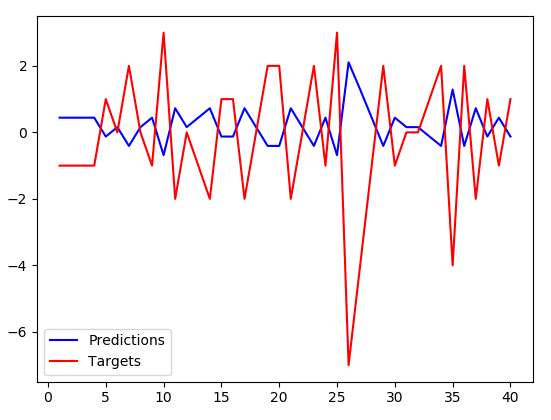While building the model, we define our cost function, which measures the value of the learned values' deviation from the predicted values. Optimizers make sure that this error reduces over the progressive epochs.

Some of the most common error functions (or cost functions ) used for regression problems are :

• Mean Squared Error ( MSE )• Root Mean Squared Deviation/Error ( RMSD/RMSE )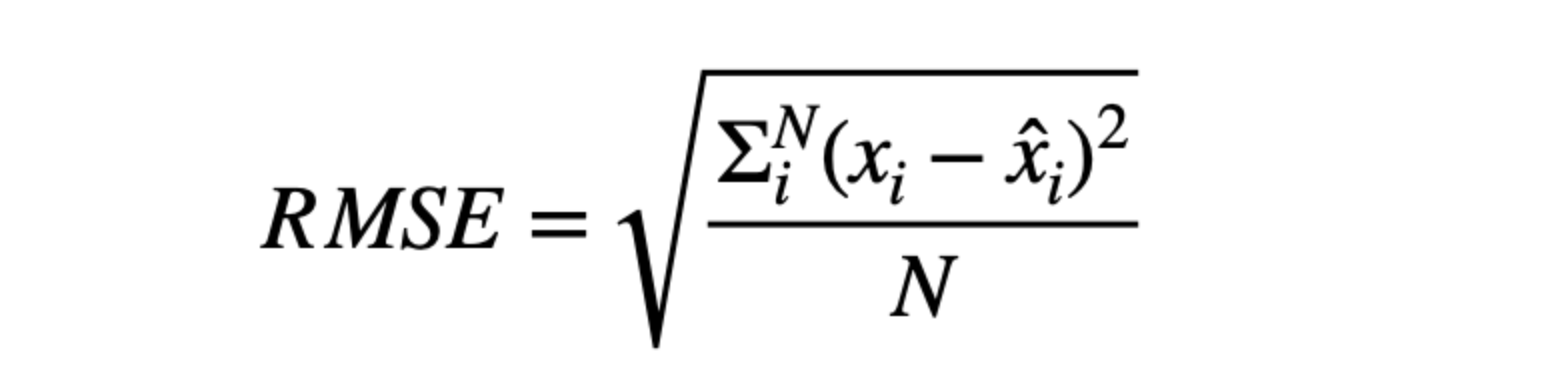• Mean Absolute Error ( MAE )Note: Xi is the predicted value, X̂i is the true value, and N is the total samples over which prediction is made.

Examples of regression problems could include:

• Predicting the price of houses based on data such as the quality of schools in the area, the number of bedrooms in the house, and the house's location.
• Predicting the sales revenue of a company based on data such as the previous sales of the company.
• Predicting the temperature of any day based on data such as wind speed, humidity, atmospheric pressure.

Algorithms for Regression:

• Linear Regression
• Support Vector Regression
• Regression Tree

### Classification Problems

In regression problems, the mapping function that algorithms want to learn is discrete. The objective is to find the decision boundary/boundaries, dividing the dataset into different categories.

More formally:

Classification is a type of problem that requires the use of machine learning algorithms that learn how to assign a class label to examples from the problem domain.

For example, suppose there are three class labels, [ Apple, Banana, Cherry]. But machines don’t have the sense to understand these labels. That’s why we need to convert these labels into a machine-readable form.
For the above example, we can define

Apple = [1,0,0], Banana = [0,1,0], Cherry = [0,0,1]

Once the machine learns from these labeled training datasets, it will give probabilities of different classes on the test dataset like this :

[P(Apple), P(Banana), P(Cherry)]

These predicted probabilities can be from one type of probability distribution function (PDF), and the actual (true) labeled dataset can be from another probability distribution function (PDF). If the predicted distribution function tends to follow the actual distribution function, we say that model is learning accurately.
Note: These PDF functions are continuous, and as a similarity between classification and regression, if predicted PDF is following the actual PDF we can say model is learning the trends.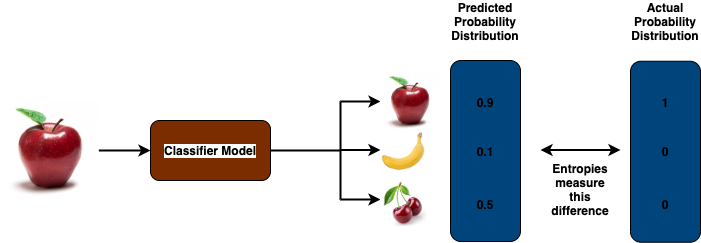Some of the common cost functions for the classification problems would be :

#### Categorical Cross-Entropy

Suppose there are M class labels, and the predicted distribution for the i-th data sample is :

P(Y) = [Yi1', Yi2', ………. , YiM’]

And, actual distribution for that sample would be,

A(Y) = [Yi1, Yi2, ……….., YiM]

Cross Entropy ( CEi) =  — (Yi1*log(Yi1') + Yi2*log(Yi2') + …… + YiM*log(YiM’))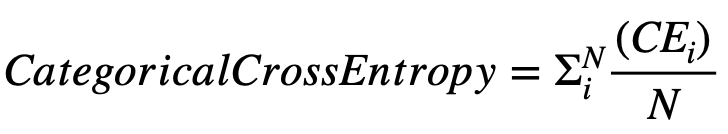#### Binary Cross-Entropy

This is a special case of categorical cross-entropy, where there is only one output that can two values, either 0 or 1. For example, if we want to predict whether a cat is present in any image or not.

Here, the cross-entropy function varies with the true value of Y,

CEi = -Yi1*log(Yi1') , if Yi1 = 1

CEi = -(1-Yi1)*log(1-Yi1'), if Yi1 = 0

And similarly, Binary-Cross-Entropy would be averaged over all the datasets.

Now, the major question that you should ask yourself,
If PDFs (probability distribution functions) are continuous in the range of [0,1], why can’t MAE/MSE be chosen here? Take a pause and think!

Reason: MAE and MSE do well when the probability of an event occurring is close to the predicted value or when the wrong prediction's confidence is not that high.

To understand the term of confidence of prediction, let’s take one example :
Suppose our ML model predicted that the patient-lady in the figure below is pregnant, and our model predicted it with the probability of 0.9. We can say that our model is very much confident. Now let’s consider one scenario when the ML model says the patient-man in the below figure is pregnant with the probability of 0.9. This is a case where the model predicts something wrong and is confident about the prediction.
To address these cases, the model needs to be penalized more for these predictions.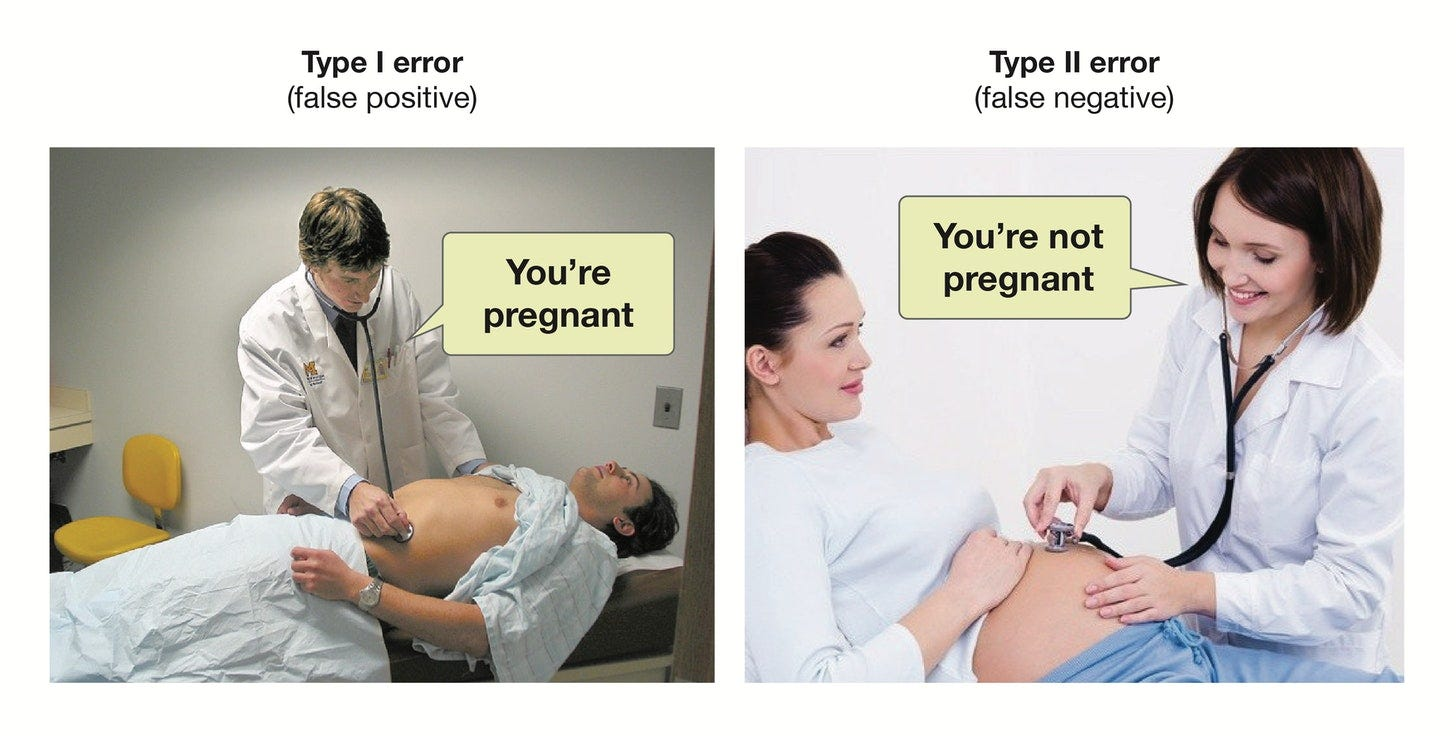Let’s calculate the cross-entropy (CE), MAE, and MSE of the case where the ML model is predicting that a man is pregnant with high confidence (Probability (Y’)= 0.8). Obviously Y = 0 here.

CE = -(1-Y)*log(1-Y’) = -(1 – 0)*log(1 – 0.8) = 1.64

MAE = |(Y-Y’) | = |0–0.8| = 0.8

MSE = |(Y-Y’)²| = (0–0.8)² = 0.64

As you can see, MAE and MSE have lower values than CE, which means the Cost function or Error function is producing more value, and hence the model should be penalized more.

That’s why we needed different cost functions for the classification problems.

To most common evaluation metric for classification models would be,

• Accuracy
• Confusion Matrix
• F1-Score
• Precision
• Recall etc.. (Definitions can be found here )

Examples of classification problems could include:

• Classifying if a mail is spam or not, based on its content, and how others have classified similar types of mails.
• Classifying a dog breed based on its physical features such as height, width, skin color.
• Classifying whether today’s weather is hot or cold.

Algorithms for Classification:

• Logistic Regression
• Support Vector Classification
• Decision Tree

### Can we solve the same problem using regression as well as classification techniques?

Yes! We can. Let’s take one example.

Problem statement: Predict steering angle of an autonomous vehicle based on the image data.

Constraints: Steering angle can take any value between -50⁰ and 50⁰ with a precision of ±5⁰.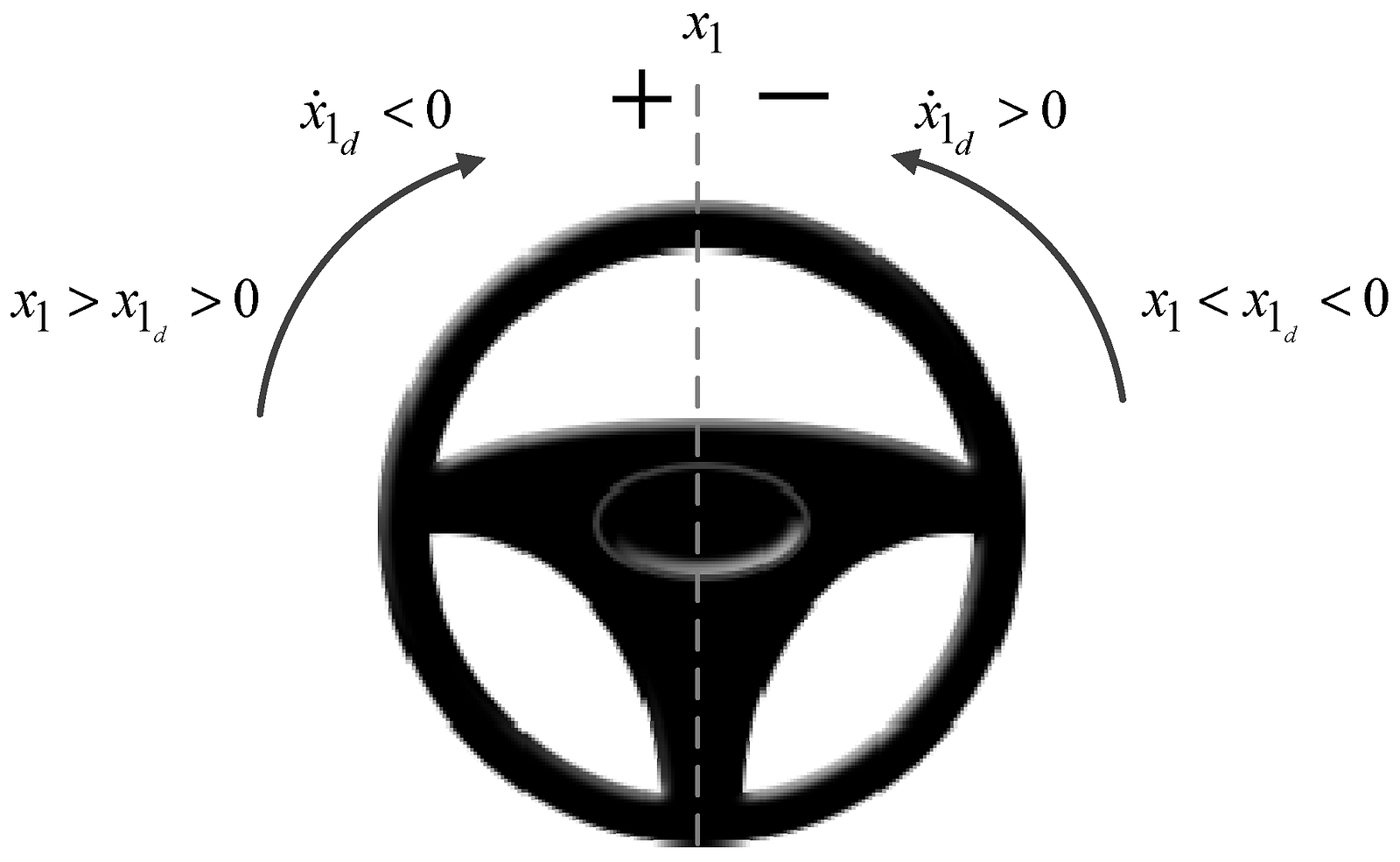Pic source: MDPI Paper

Regression Solution: This solution is simple, where we can map the images to the steering angle's continuous function, which continuously gives the output.

Classification Problem: Precision is ±5⁰, so we can divide the entire range of -50⁰ to 50⁰ in 20 different classes by grouping every 5⁰ at a time. This way, the problem is converted into a classification problem.

### Critical Questions to explore

1. What is the difference between classification and regression problems?
2. Why do we have different cost functions in case of different problem statements?
3. How do these cost functions decide that the problem is a classification problem or a regression problem?
4. When do we use binary cross-entropy and when do we use categorical cross-entropy?
5. Can you find more such problem statements that can be solved via both ways?

### Conclusion

In this article, we have described the concepts of classification and regression problems in detail. We discussed the difference in cost functions like MAE, MSE, and Categorical Cross Entropies that are the key difference at the time of model building using modern frameworks like Tensorflow, Keras, or PyTorch. Meanwhile, in the end, we discussed a common problem statement where we discussed one famous problem statement which can be solved by considering the problem statement as classification as well as a regression problem statement. We hope you have enjoyed the article and learned something new.

### Enjoy Thinking! Enjoy Learning! Enjoy Algorithms!

Get well-designed application and interview centirc content on ds-algorithms, machine learning, system design and oops. Content will be delivered weekly.